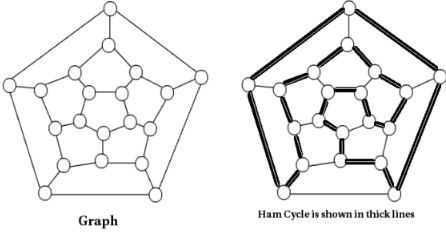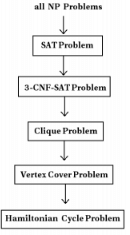Hamiltonian Cycle Problem

# Hamiltonian Cycle Problem - Theory of Computation - Computer Science Engineering (CSE)

Part VII. Hamiltonian Cycle Problem

11 Hamiltonian Cycle Problem

Hamiltonian Cycle
A hamiltonian cycle in an undirected graph is a simple cycle which contains every vertex of the graph.
A graph which contains a hamiltonian cycle is called hamiltonian. A graph which does not contain a hamiltonian cycle is called nonhamiltonian.
Example:
Consider the following graph:Note that the hamiltonian cycle path passes through every vertex.

Hamiltonian Cycle Problem
The hamiltonian cycle problem is to check whether a graph has a hamiltonian cycle..

Hamiltonian Cycle Problem is NP-Complete
We know that a problem is in class NP-Complete if: it is in NP and it is NP Hard.

Theorem:
Hamiltonian cycle problem is NP-complete.

Proof:

Step 1: Prove that hamiltonian cycle problem is in class NP.
A set with n elements has 2n possible subsets. Then a graph with n vertices has 2n possible permutations of vertices.So an algorithm needs to check whether any one of these permutation form a hamiltonian path. If the graph is represented as an adjacency matrix, it will take n! comparisons. It has worst case time complexity Θ(2√n). (not polynomial time, but exponential time).
Let we are given a graph and a ’certificate’ telling that a sequence of vertices form a hamiltonian cycle. An algorithm can verify whether this path is hamiltonian in polynomial time.
So hamiltonian cycle problem is in class NP.

Step 2: Prove that hamiltonian cycle problem is NP-hard.
We know that a problem is NP-Hard, if every problem in NP can be reduced to this problem in polynomial time.
The approach we used is as follows:
In the previous section, we already proved that all NP problems can be reduced to SAT. Then, again we found that SAT problem can be reduced to 3-CNF-SAT problem. Again, we reduced 3-CNF-SAT problem to clique problem. again, we reduced clique problem to vertex cover problem. Then, if we can reduce vertex cover problem to hamiltonian cycle problem in polynomial time, we can say that hamiltonian cycle problem is NP-hard.We need to reduce vertex cover problem to hamiltonian cycle problem.

The document Hamiltonian Cycle Problem | Theory of Computation - Computer Science Engineering (CSE) is a part of the Computer Science Engineering (CSE) Course Theory of Computation.
All you need of Computer Science Engineering (CSE) at this link: Computer Science Engineering (CSE)

## Theory of Computation

18 videos|56 docs|44 tests

## FAQs on Hamiltonian Cycle Problem - Theory of Computation - Computer Science Engineering (CSE)

 1. What is the Hamiltonian Cycle Problem in Computer Science Engineering (CSE)?Ans. The Hamiltonian Cycle Problem in CSE is a well-known problem in graph theory that involves finding a cycle in a graph that visits every vertex exactly once. In other words, it seeks to determine whether there exists a path in a graph that visits each vertex exactly once, starting and ending at the same vertex.
 2. Why is the Hamiltonian Cycle Problem important in CSE?Ans. The Hamiltonian Cycle Problem is important in CSE because it has various real-world applications. For example, it can be used in optimizing routing problems, designing computer networks, solving scheduling problems, and analyzing DNA sequences. Its significance lies in its ability to find efficient solutions to problems that can be modeled as graphs.
 3. What is the complexity of the Hamiltonian Cycle Problem?Ans. The Hamiltonian Cycle Problem is known to be an NP-complete problem, which means that there is no known polynomial-time algorithm to solve it for all inputs. It is a computationally challenging problem, and researchers have focused on developing approximation algorithms and heuristics to find near-optimal solutions.
 4. How can the Hamiltonian Cycle Problem be solved in practice?Ans. The Hamiltonian Cycle Problem can be solved using various algorithms, such as the brute-force approach, backtracking, dynamic programming, and branch and bound. However, these algorithms may not be efficient for large graphs due to the exponential time complexity. In practice, heuristics and approximation algorithms are often used to find good solutions within a reasonable time frame.
 5. Can the Hamiltonian Cycle Problem be solved in polynomial time for specific cases?Ans. Yes, for certain types of graphs, the Hamiltonian Cycle Problem can be solved in polynomial time. For example, for complete graphs (graphs where every pair of vertices is connected by an edge), a Hamiltonian cycle always exists, and finding it can be done in O(n!) time complexity. Additionally, for graphs with a specific structure or constraints, specialized algorithms can be devised to solve the problem more efficiently. However, in general, the problem remains NP-complete.

## Theory of Computation

18 videos|56 docs|44 testsExplore Courses for Computer Science Engineering (CSE) examSignup to see your scores go up within 7 days! Learn & Practice with 1000+ FREE Notes, Videos & Tests.
10M+ students study on EduRev
Track your progress, build streaks, highlight & save important lessons and more!
Related Searches

,

,

,

,

,

,

,

,

,

,

,

,

,

,

,

,

,

,

,

,

,

;